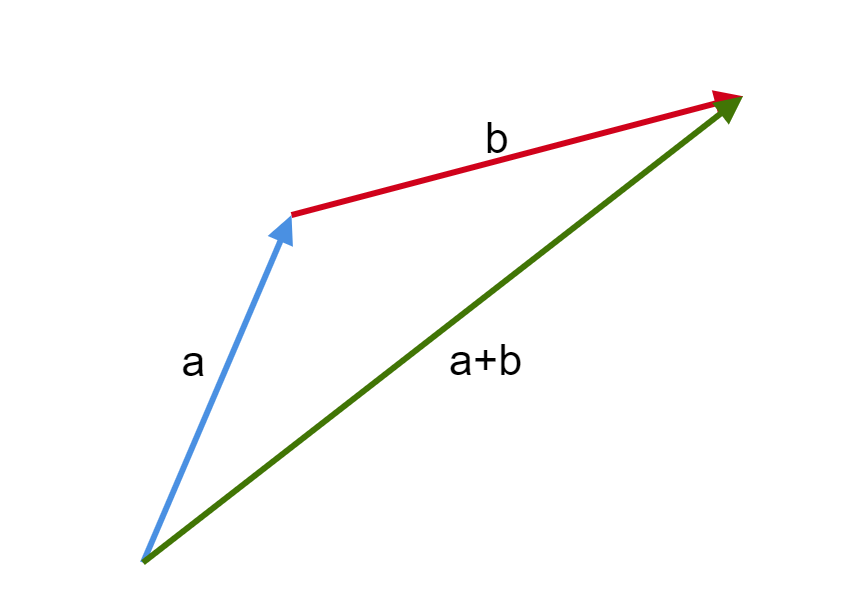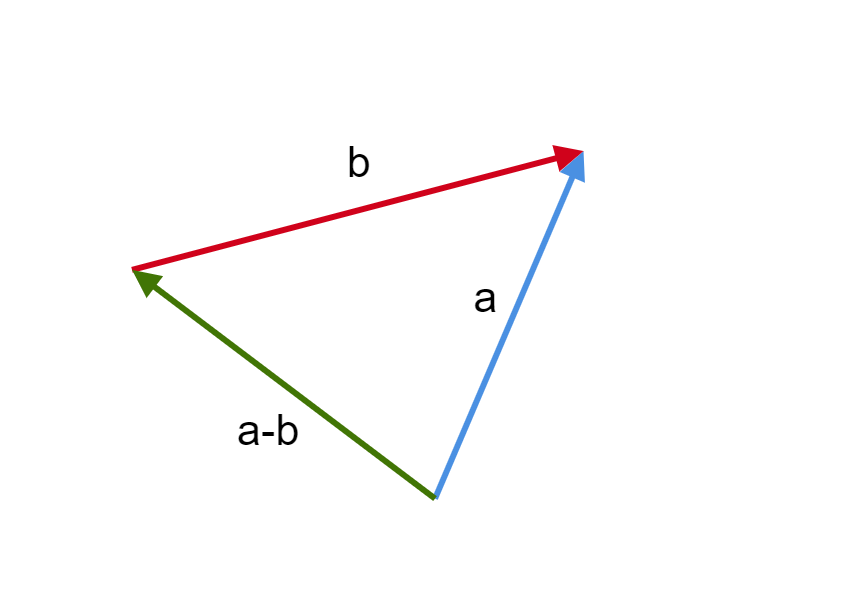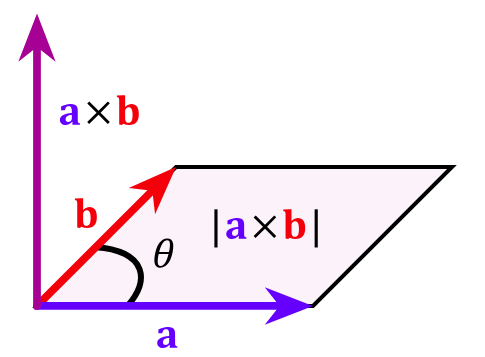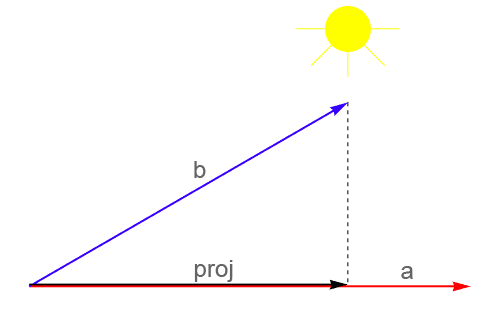# Vector Calculator

Created by Anna Szczepanek, PhD
Reviewed by Dominik Czernia, PhD candidate and Jack Bowater
Last updated: Sep 29, 2022

Welcome to our vector calculator! It'll help you perform and understand a whole bunch of vector operations. Do you have the Cartesian coordinates of two vectors, or just know their vector direction and magnitude? Maybe you need to find the vector between two points? This vector calculator can deal with all those situations; it performs:

• Vector subtraction;
• Vector multiplication (both cross product and dot product!); and
• Vector projections.

As a bonus, we'll also teach you what the norm of a vector is and how to normalize a vector.

## Cartesian coordinates

In a rectangular (called Cartesian) xy-coordinate system, we describe vector a in a 2D plane by its Cartesian coordinates:

a = [ax, ay].

These coordinates correspond to the fact that we can decompose a displacement along vector a into horizontal displacement ax along x-axis and vertical displacement ay along the y-axis.

Similarly, we describe vectors in 3D-space using the Cartesian xyz-system with three numbers:

a = [ax, ay, az],

which correspond to displacements along the x-, y-, and z-axis, respectively.

## Vector direction and magnitude

We can also describe a plane vector in terms of vector direction and magnitude. The magnitude of a vector is its length (also called the norm) and the direction of a vector is the angle between the horizontal axis and the vector.

Let [ax, ay] be the Cartesian coordinates of a vector with magnitude m and direction θ. To convert one set of coordinates to the other, use the following formulas:

ax = m × cos(θ)

ay = m × sin(θ)

## How to determine a vector between two points?

If you need to determine a vector between two points, i.e., from the initial and final point, just subtract the coordinates of the final point from the initial point:

• Initial point (origin): a = [ax, ay, az]

• Final point (tip/head): b = [bx, by, bz]

• Vector between these two points:

[bx - ax, by - ay, bz - az]

Let's have a look at an example:

• Initial point: [1, 2, 3]

• Final point: [1, 1, -1]

• Vector between these two points:

[1 - 1, 1 - 2, 3 - (-1)] = [0, -1, 4]

## How to normalize a vector? What is the norm of a vector?

Vector normalization is just squeezing/stretching a vector so that it has unit magnitude. Remember that a vector's direction must be kept!

To normalize a vector, find its norm using the Pythagorean theorem. The norm of a vector is its magnitude: the square root of the sum of the squared coordinates of your vector. Then divide each coordinate of the initial vector by this norm. You may also want to use the vector magnitude calculator to find the norm, which is the simpler version of this vector calculator.

Example:

Let a = [2, 3, 4]. Let us find the norm of a:

|a| = √(4 + 9 + 16) = √29.

Normalization of a gives us the vector a/|a| = [2/√29, 3√29, 4/√29]. Do you know that we have a dedicated calculator for normalizing vectors?

## How to use this vector calculator?

To use the vector calculator, simply follow the steps below:

1. Tell us whether you are working with plane (2D) or space (3D) vectors.
2. Decide on the vector operation you want to perform. You can choose vector addition or subtraction, vector multiplication (dot or cross product), normalization, vector projection, or finding the vector between two points.
3. Enter your data. You may choose between Cartesian coordinates or vector direction & magnitude in the case of plane vectors.
4. Our vector calculator returns the results immediately. Enjoy! 😊

• In Cartesian coordinates, we can perform vector addition simply by adding the corresponding components of the vectors:

for a = [ax, ay, az],

and b = [bx, by, bz],

we have a + b = [ax + bx, ay + by, az + bz].

Example:

The sum of a = [2, 3, 4] and b = [1, -2, 3] is:

a + b = [2 + 1, 3 + (-2), 4 + 3] = [3, 1, 7]

• Graphical method: for two vectors a and b, if we want to obtain the vector sum a + b, place the origin of b to the tip of a. The resulting vector goes from the origin of a to the tip of b. We call this rule the parallelogram law:## Vector subtraction

The subtraction of vector b from vector a is just the addition of -b to a. To find vector -b, take the coordinates of b with opposite signs; change pluses to minuses and minuses to pluses:

if b = [1, -2, 4],

then -b = [-1, 2, -4]

• Consequently, in Cartesian coordinates, we perform vector subtraction a - b by subtracting the coordinates of b from those of a:

if a = [ax, ay, az]

and b = [bx, by, bz],

then a - b = [ax - bx, ay - by, az - bz].

Example:

The difference of a = [2, 3, 4] and b = [1, -2, 3] is given by

a - b = [2 - 1, 3 - (-2), 4 - 3] = [1, 5, 1].

• Graphical method: we obtain the vector difference a - b by placing the tip of b to the tip of a and drawing a vector from the origin of a to the origin of b:## Vector multiplication

Be careful when asked to multiply vectors - there are several different types of vector multiplication! The most popular are the cross product and dot product, which we describe below:

#### Vector multiplication - Cross product

The cross product is an operation denoted by the operator × and takes two vectors and returns another vector.
The formula is as follows:

a × b = |a| * |b| * sin(θ) * n,

where:

• θ is the angle between a and b;
• |a| and |b| are their respective magnitudes; and
• n is the unit vector perpendicular to both a and b, determined by the Right Hand Rule.

Right Hand Rule:

Position your right hand so that your index finger points along vector a and your middle finger points along vector b: your thumb shows the direction of the cross product a × b.

• Graphical interpretation: The resulting vector a × b is at a right angle (perpendicular) to the initial vectors and its magnitude is equal to the area of a parallelogram spanned by the initial vectors:• In terms of Cartesian coordinates:

for a = [ax, ay, az],

and b = [bx, by, bz], we have

a × b = [ay×bz - az×by, az×bx - ax×bz, ax×by - ay×bx].

Example:

The cross product of a = [2, 3, 4] and b = [1, -2, 3] is equal to

a × b = [3 × 3 - 4 × (-2), 4 × 1 - 2 × 3, 2 × (-2) - 3 × 1] = [17, -2, -7].

• Yes, the formula looks a bit intimidating. It is easier to remember it once you realize that the coordinates of the product are the determinants of the appropriate 2 x 2 matrices:

1. The first coordinate is1. The second coordinate is1. The third coordinate is• Be careful with the order of vectors, because, in contrast to the dot product, the order matters for the cross product! More precisely, we have b × a = - a × b, so, if you had the order wrong, just change the sign and you'll be all right 🙃

• The vector cross product has lots of applications in physics & engineering - e.g., you can use to determine the Lorentz force. Go check our cross product calculator to find out more!

#### Vector multiplication - Dot product

The dot product (also known as the scalar product) is an operation denoted by the operator · that takes two vectors and returns a number. For two vectors a and b, their dot product is the product of their magnitudes (norms) |a| and |b| and the cosine of the angle θ between them:

a · b = |a| * |b| * cos(θ).

• In Cartesian coordinates, the dot product is the sum of the products of the corresponding coordinates of your two vectors:

a · b = ax * bx + ay * by + az * bz

Example:

The dot product of a = [2, 3, 4] and b = [1, -2, 3] is

a · b = 2 * 1 + 3 * (-2) + 4 * 3 = 2 - 6 + 12 = 8.

• As you may have already concluded from the formula, order does not matter here: a · b = b · a.

• Tip: if you calculate the dot product of a vector with itself, you arrive at a squared magnitude of the vector: a · a = |a|²!

• For more details, check our dedicated dot product calculator.

## Vector projection

The projection of b onto a is the vector which is the best approximation of b among the vectors obtained by stretching and squeezing vector a. Hence, to find the projection, you just need to know the proper stretching/squeezing factor.

• Formula:

The projection of b on a is vector a scaled by:

a · b / |a|².

Example:

Let a = [2, 3, 4] and b = [1, -2, 3]. Let's calculate the projection of b onto a. First, let's find the scaling factor. We have calculated above that a · b = 8 and |a| = √29. Consequently, the projection of b onto a is:

8/29 * [2, 3, 4] = [16/29, 8/87, 32/29]

• To find the projection of b onto a graphically, you need to decompose b along the axises spanned by a and perpendicular to a. The component that lies alongside a is the projection of b onto a. You may also think of this as the shadow that vector b would cast on vector a if there were a light source hung above these vectors:To learn more about projecting vectors onto other vectors, go to our dedicated vector projection calculator.

## FAQ

### What is a vector?

A vector is a mathematical object defined by:

• A magnitude; and
• A direction.

They are substantially different than scalar quantities: the latter doesn't change with the direction.

Vectors have widespread applications in physics, where they fit quantities such as displacement and velocity.

### How do I find the projection of a vector onto another?

If you consider the vectors a and b, you can find the projection of a onto b by following the next steps:

1. Calculate the dot product between a and b: a · b.
2. Calculate the dot product of b by itself: b · b.
3. Calculate the ratio between the two results: (a · b)/(b · b).
4. Multiply the result (a scalar) by the vector b: [(a · b)/(b · b)] × b.

### What is the scalar product of two vectors?

The scalar product of two vectors is an operation that consists of summing the product of the components of two vectors "dimension-wise".

If you have two vectors, a = (a₁, a₂, a₃, ..., aₙ) and b = (b₁, b₂, b₃, ..., bₙ), follow these two steps to compute the scalar product:

1. Calculate the product of each pair of components: a₁ × b₁, a₂ × b₂, etc.
2. Sum the results: (a₁ × b₁) + (a₂ × b₂).

Notice that you can only compute the scalar product of vectors with the same number of components.

### What is the magnitude of a vector with components (3,1,4,1,5)?

To compute the magnitude of the vector with components (3,1,4,1,5), apply the generalized Pythagorean theorem over all the components. If the vector is a = (a₁, a₂, a₃, ..., aₙ), we found the magnitude with the formula:
||a||= √(a₁² + a₂² + a₃² + ... + aₙ²)
In the case of the vector (3,1,4,1,5), the magnitude is:
√(3² + 1² + 4² + 1² + 5²) = √(9+1+16+1+25) = √(52) ≈ 7.21

Anna Szczepanek, PhD
Vectors in
2D
Operation
Perform vector addition a + b.
Vector a:
Cartesian coordinatesx
y
Vector b:
Cartesian coordinates
x
y
Result
Vector a + b:
Cartesian coordinates
x
y
People also viewed…

### Arc length

Our arc length calculator can calculate the length of an arc of a circle and the area of a sector.

### Circumference

Use this free circumference calculator to find the area, circumference and diameter of a circle.

### Humans vs vampires

Vampire apocalypse calculator shows what would happen if vampires were among us using the predator - prey model.

### Sin triangle

The sine triangle calculator is a tool that will help you determine the sine of any angle given in degrees, radians, or pi radians.